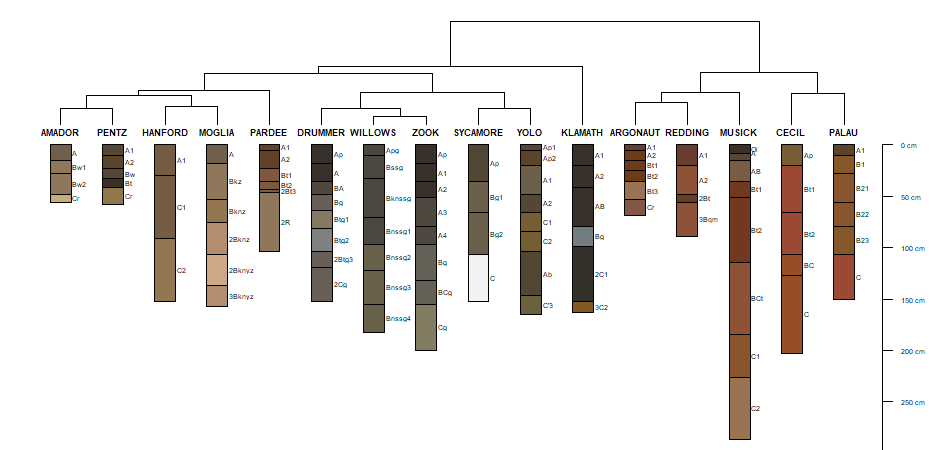This content has been moved to http://ncss-tech.github.io/stats_for_soil_survey/book2/ordination.html

# 1 Introduction

Nearly every aspect of soil survey involves the question: “Is X more similar to Y or to Z?” The quantification of similarity within a collection of horizons, pedons, components, map units, or even landscapes represents an exciting new way to enhance the precision and accuracy of the day-to-day work of soil scientists. After completing this module, you should be able to quantitatively organize objects based on measured or observed characteristics in a consistent and repeatable manner. Perhaps you will find a solution to the long-standing “similar or dissimilar” question.

## 1.1 Objectives

• Learn essential vocabulary used in the field of numerical taxonomy. Review some of the literature.
• Gain experience with R functions and packages commonly used for clustering and ordination.
• Learn how to create and interpret a distance matrix and appropriate distance metrics.
• Learn how to create and interpret a dendrogram.
• Lean the basics and application of hierarchical clustering methods.
• Lean the basics and application of partitioning clustering methods.
• Learn the basics and application of ordination methods.
• Apply skills to a range of data sources for soils and vegetation.
• Apply techniques from numerical taxonomy to addressing the “similar or dissimilar” question.
• Learn some strategies for coping with missing data.

# 2 Whirlwind Tour

Most of the examples featured in this whirlwind tour are based on soil data from McGahan, D.G., Southard, R.J, Claassen, V.P. 2009. Plant-available calcium varies widely in soils on serpentinite landscapes. Soil Sci. Soc. Am. J. 73: 2087-2095. These data are available in the dataset “sp4” that is built into aqp package for R.

## 2.1 Similarity, Disimilarty, and Distance

There are shelves of books and thousands of academic articles describing the theory and applications of “clustering” and “ordination” methods. This body of knowledge is commonly described as the field of numerical taxonomy (Sneath and Sokal 1973). Central to this field is the quantification of similarity among “individuals” based on a relevant set of “characteristics.” Individuals are typically described as rows of data with a single characteristic per column, together referred to as a data matrix. For example:

name clay sand Mg Ca CEC_7
A 21 46 25.7 9.0 23.0
ABt 27 42 23.7 5.6 21.4
Bt1 32 40 23.2 1.9 23.7
Bt2 55 27 44.3 0.3 43.0

Quantitative measures of similarity are more conveniently expressed as distance, or dissimilarity; in part because of convention and in part because of computational efficiency. In the simplest case, dissimilarity can be computed as the shortest distance between individuals in property-space. Another name for the shortest linear distance between points is the Euclidean distance. Evaluated in two dimensions (between individuals $$p$$ and $$q$$), the Euclidean distance is calculated as follows:

$D(p,q) = \sqrt{(p_{1} - q_{1})^{2} + (p_{2} - q_{2})^{2}}$

where $$p_{1}$$ is the 1st characteristic (or dimension) of individual $$p$$.

There are many other ways to define “distance” (e.g. distance metrics), but they will be covered later.

Using the sand and clay percentages from the data above, dissimilarity is represented as the length of the line connecting any two individuals in property space.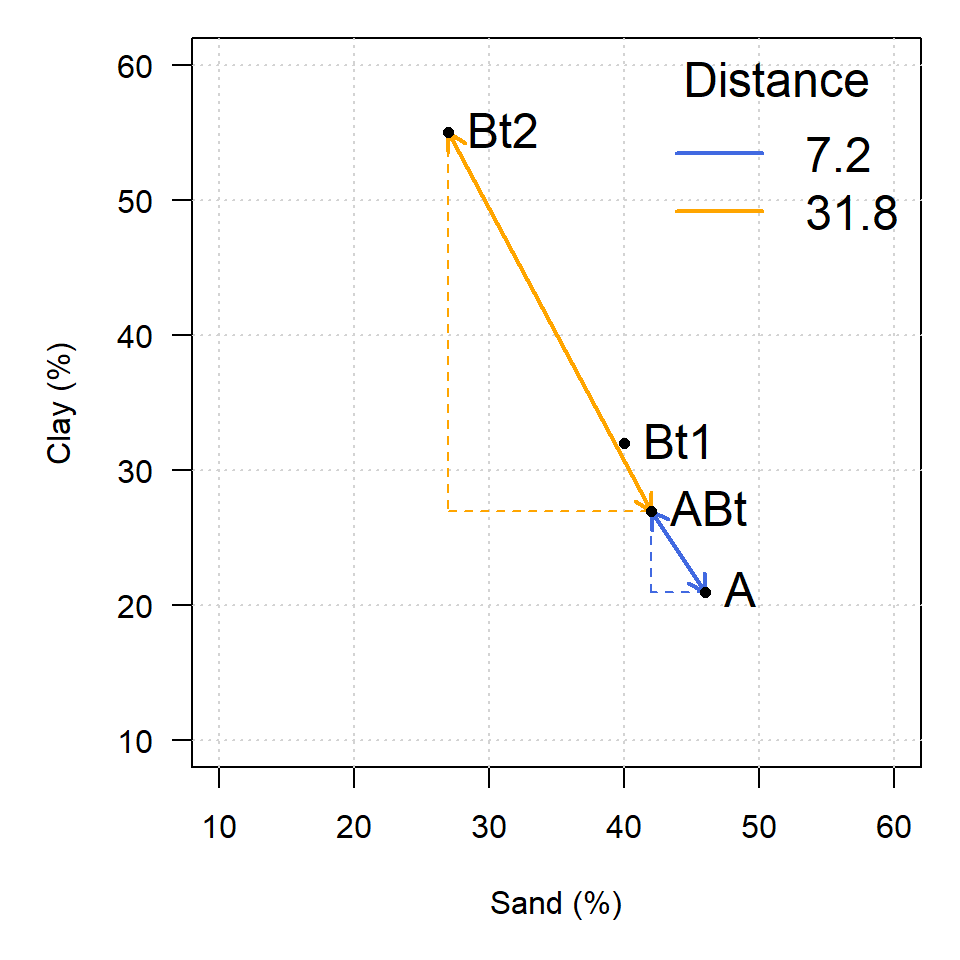The following is a matrix of all pair-wise distances (the distance matrix):

A ABt Bt1 Bt2
A 0.0 7.2 12.5 38.9
ABt 7.2 0.0 5.4 31.8
Bt1 12.5 5.4 0.0 26.4
Bt2 38.9 31.8 26.4 0.0

Note that this is the full form of the distance matrix. In this form, zeros are on the diagonal (i.e. the distance between an individual and itself is zero) and the upper and lower “triangles” are symmetric. The lower triangle is commonly used by most algorithms to encode pair-wise distances.

A ABt Bt1
ABt 7.2
Bt1 12.5 5.4
Bt2 38.9 31.8 26.4

Interpretation of the matrix is simple: Individual “A” is more like “ABt” than like “Bt1.” It is important to note that quantification of dissimilarity (distance) among individuals is always relative: “X is more like Y, as compared to Z.”

### 2.1.1 Distances You Can See: Perceptual Color Difference

Simulated redoximorphic feature colors, constrast classes and CIE $$\Delta{E_{00}}$$. Details here.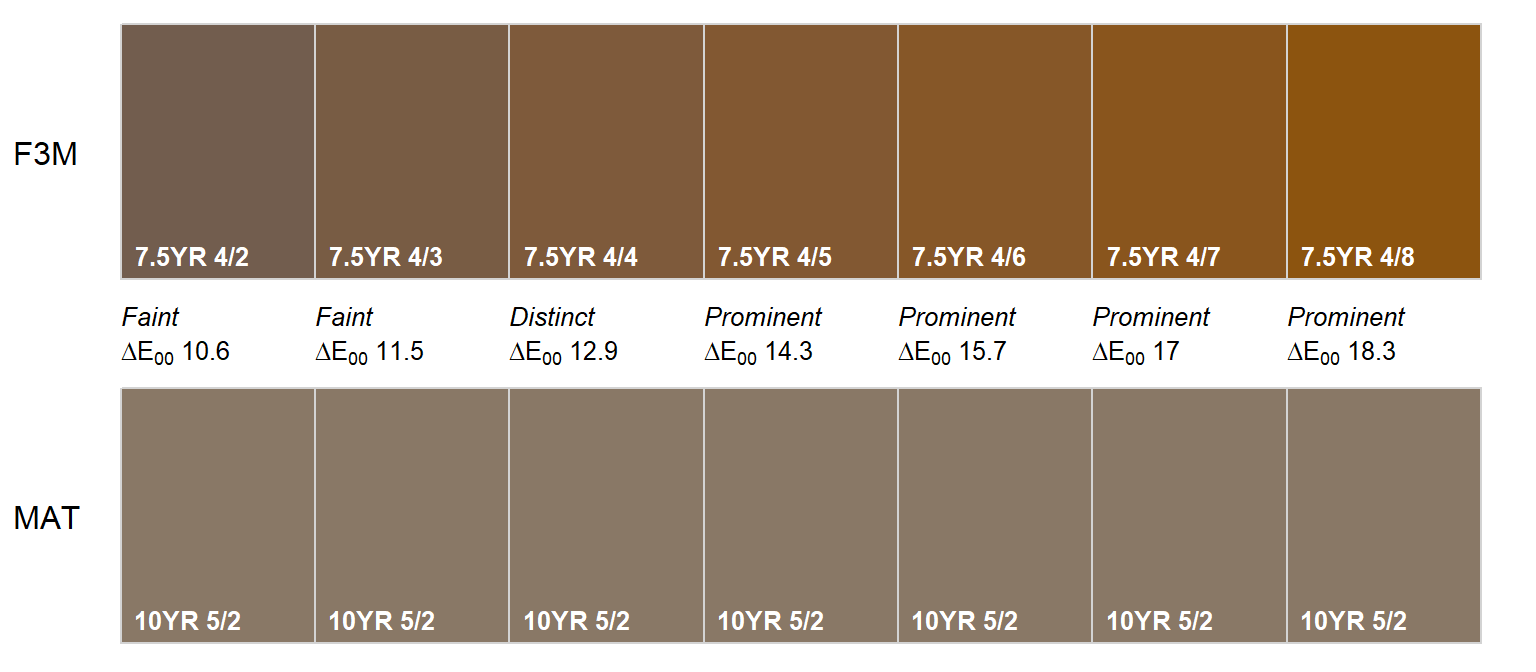## 2.2 Standardization of Characteristics

Euclidean distance doesn’t make much sense if the characteristics do not share a common unit of measure or range of values. Nor is it relevant when some characteristics are categorical and some are continuous. For example, distances are distorted if you compare clay (%) and exchangeable Ca (cmol/kg).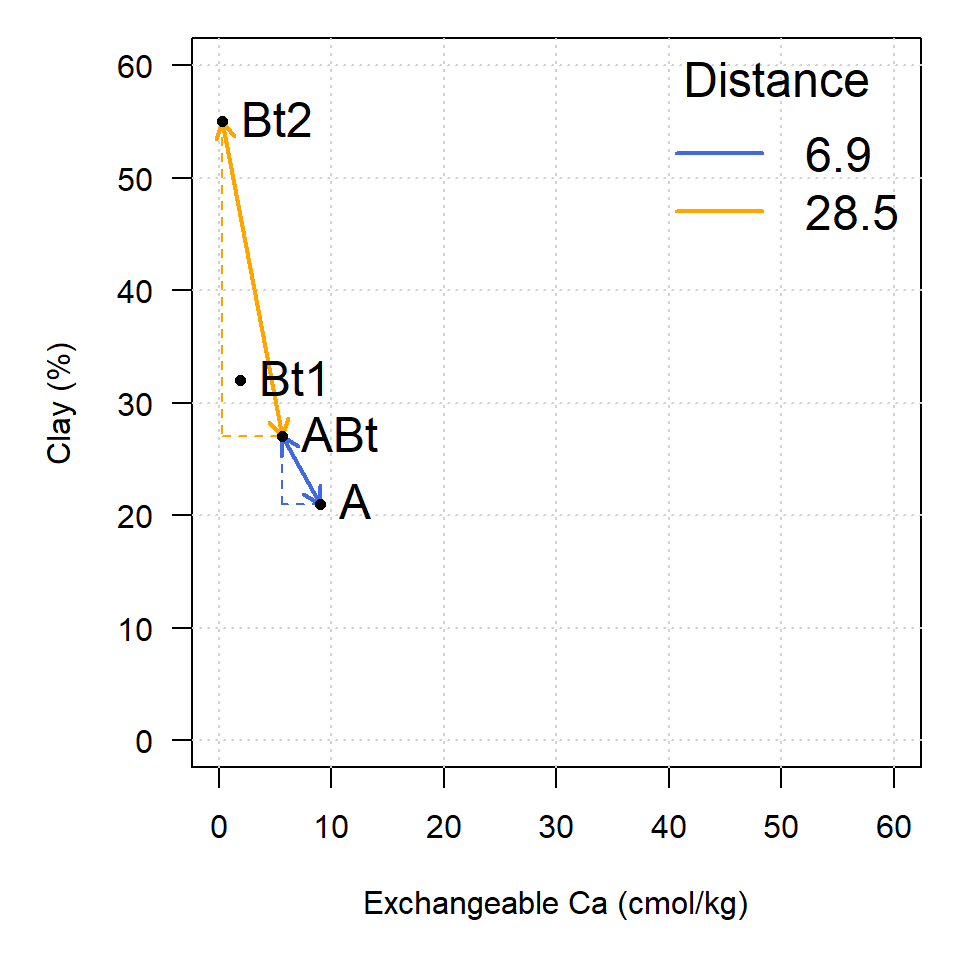In this example, exchangeable Ca contributes less to the distance between individuals than clay content, effectively down-weighting the importance of the exchangeable Ca. Typically, characteristics are given equal weight (Sneath and Sokal 1973); however, weighting is much simpler to apply after standardization.

Standardization of the data matrix solves the problem of unequal ranges or units of measure, typically by subtraction of the mean and division by standard deviation (z-score transformation).

$x_{std} = \frac{x - mean(x)}{sd(x)}$

There are several other standardization methods covered later. The new data matrix looks like the following:

name clay sand Mg Ca CEC_7
A -0.86 0.88 -0.35 1.23 -0.47
ABt -0.45 0.40 -0.55 0.36 -0.63
Bt1 -0.12 0.15 -0.60 -0.59 -0.40
Bt2 1.43 -1.43 1.49 -1.00 1.49

Using the standardized data matrix, distances computed in the property space of clay and exchangeable calcium are unbiased by the unique central tendency or spread of each character.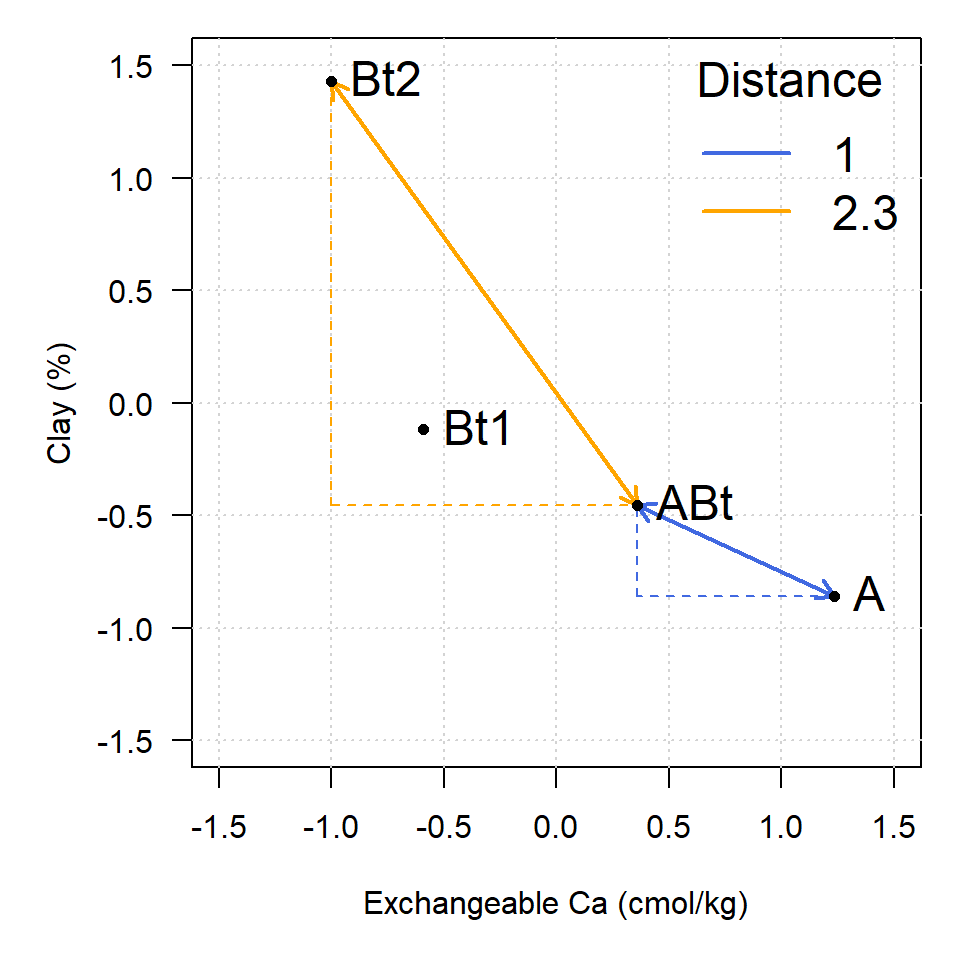Rarely can the question of “dissimilarity” be answered with only two characteristics (dimensions). Euclidean distance, however, can be extended to an arbitrary number of $$n$$ dimensions.

$D(p,q) = \sqrt{ \sum_{i=1}^{n}{(p_{i} - q_{i})^{2}} }$

In the equation above, $$i$$ is one of $$n$$ total characteristics. Imagining what distance “looks like” is difficult if there are more than three dimensions. Instead, examine the distance matrix calculated using all five characteristics.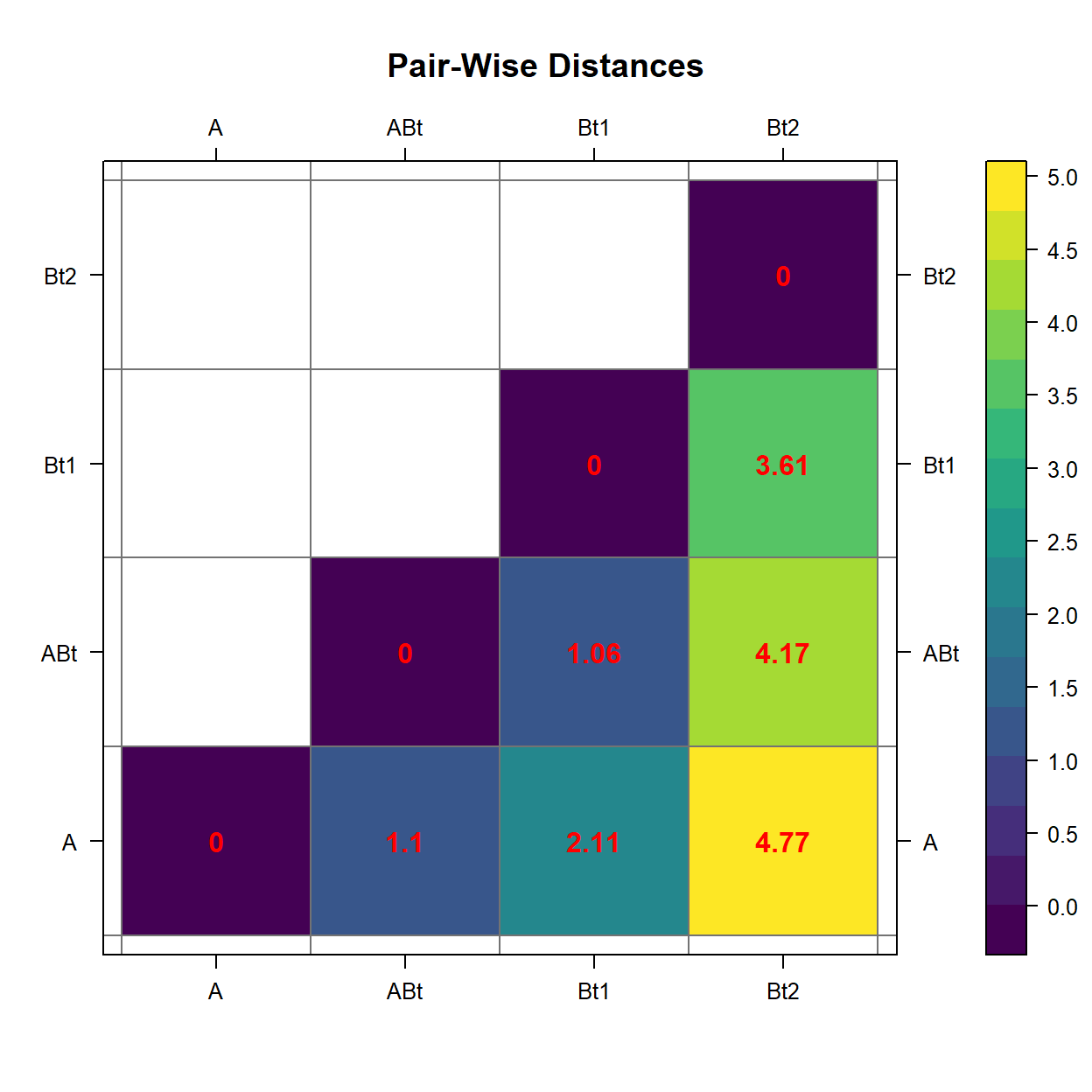Rescaling to the interval {0,1}.